Euler's formula for eiθA geometric riff on an analytic theme

Scott E. Brodie

 'Gentlemen, that is surely true, it is absolutely paradoxical; we cannot understand it, and we don't know what it means. But we have proved it, and therefore we know it must be the truth.' Benjamin Peirce

An intuitive understanding of Euler's formula for the complex exponential,

e = cos θ + i sin θ,

remains elusive, notwithstanding hundreds of years of contemplation. While the formula can be "motivated" or "justified" by rearrangement of the infinite series for the sine, cosine, and exponential functions, this argument requires a deeper understanding of the theory of infinite series than is likely available when Euler's formula is first encountered. A persuasive argument can also be had by demanding that differentiation of the complex exponential obey the chain rule, which leads to simple differential equations whose unique solutions recover Euler's formula [Allendoerfer], but, again, one must appeal to results from a more advanced course.

In this note, I suggest a simple heuristic motivation for Euler's formula which requires only simple geometry and limiting arguments which are within reach of a well-informed high-school student. While the argument cannot be called a "proof," for reasons which are indicated below, the construction is highly suggestive, and perhaps explains Euler's formula in a more concrete way than those based purely on analytic reasoning.

The number e is ordinarily defined by the limit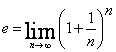This definition for e as a limit implies a simple formula for the powers of e, which is surely valid at least for positive real exponents:

 (*)where the first step follows from the continuity of the power function, and the second step is obtained by the substitution m = np, n = m/p, or 1/n = p/m, since m = np goes to infinity whenever n does.

While, on its face, the first line in (*) makes little sense if we take p = , the third line in (*) is just the limit of a sequence of complex numbers, each of which is simply a complex number raised to an integer power -- this format avoids the uninterpretable imaginary exponent.

In fact, we show below that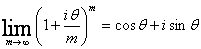As an added benefit, we can apply the geometric interpretation of complex number arithmetic to visualize each term of the sequence. The magnitude of the mth term is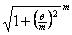,

while the argument (the angle from the positive x-axis) of the mth term is

m tan-1 (θ / m).

The problem with this program as a "proof" of the Euler equation is the substitution step in (*). In the case of a positive real exponent p, the sequence of values of m = np approaches infinity in the same sense as the original sequence of indices n, and the usual definition of the limit of a sequence applies without difficulty. This of course is not true for a sequence of the form

m = inθ

as the definition of the limit of a sequence (for each ε > 0there exists an M such that m > M implies ...) no longer makes sense - the complex numbers are not ordered.

In order to visualize the approach to the limit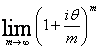consider an explicit example, say θ = 2 (Figure 1.)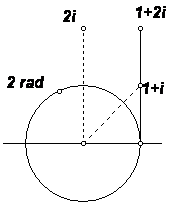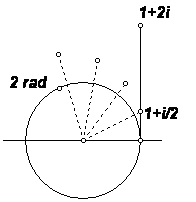Figure 1

The first element of the sequence (1 + 2i/n)n is 1 + 2i, which corresponds to the point (1, 2). This point, and the second element of the sequence, (1 + i)2 = 2i, are shown in the left-hand panel of the figure. The right hand panel illustrates the construction of the 4th element of the sequence, (1 + i/2)4. The magnitude of the factor 1 + i/2 is slightly greater than 1.12; the argument is tan-1(1/2), about 26.6° or 0.46 radians. The magnitude of the 4th power is about 1.56; the argument of the 4th power is about 106.3°, or approximately 1.86 radians.

A similar plot of the construction of (1 + 2i/10)10is shown in Figure 2: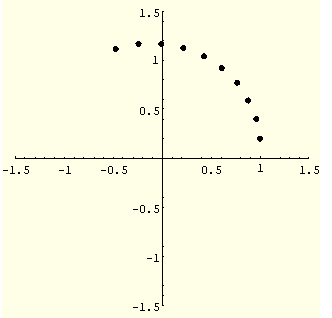Figure 2

Evidently, as n increases, the successive points of the form (1 + 2i/n)k more and more closely approach the unit circle. With increasing n, the argument of the nth power appears to approach 2 radians. The nature of the approach to the limit is illustrated in the Figure 3., which shows a plot of the first 30 terms of the sequence (1 + 2i/n)n. (Each dot in this figure corresponds to the "last" dot of a figure similar to the ones above):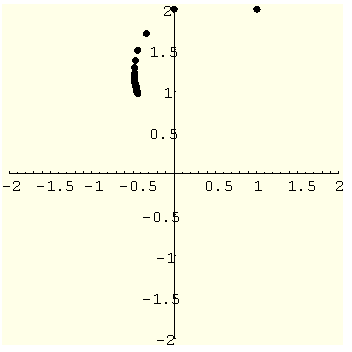Figure 3

In other words, with increasing n, the points (1 + 2i/n)k, (k = 1 to n), appear to ever more closely "wrap" around the unit circle, approaching as a limit the point on the circle at an angle of 2 radians from the positive real axis. But the identity of this point is well known: with Cartesian coordinates of (cos 2, sin 2), it is the complex number cos 2 + isin 2. Indeed, the approach to this limit realizes in a concrete way the "wrapping function" of elementary trigonometry!

Of course, there is nothing special about the value θ = 2. In order to prove the result in the general case, it is convenient to analyze the magnitude and the argument of the limit point separately.

First, consider the magnitude. We must show that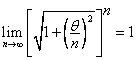There is no point in retaining the square-root: we may as well show that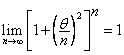,

since a sequence of positive real numbers and the sequence of its square roots approach 1,or not,together.

First, it let's put the exponent back on the outside of the square brackets:Thus, we need only show that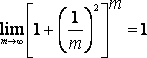To this end, expand in terms of the binomial theorem:Of course,,

so taking the limit as m increases without bound, we have,

QED.

(This calculation can be greatly simplified by the use of logarithms, but that seems contrary to the spirit of this exercise.)

Now consider the argument of the limit point. We must show that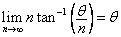The geometry is shown in the figure: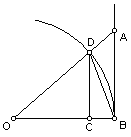O is the origin; B is the point (1,0), and A is the point

1 + / n

Denote the angle DOB by θn. Then tan θn = θ/n, or θn = tan-1 (θ/n). Now compare the areas of triangle DOB = ½ sin θn , sector DOB = ½ θn , and triangle AOB = ½ tan θn. By inclusion, after canceling a common factor of ½, we have

sin θn< θn< tan θn.

Now sin θn= CD. Since triangle DCO is similar to triangle ABO, we have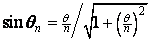.

Thus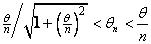,

since tan θn = θ/n.

Now multiply through by n: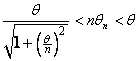.

Now take the limit as n increases without bound: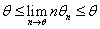,

or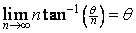,

QED.

Reference

1. Allendoerfer CB, Editorial - The Proof of Euler's Equation. American Mathematical Monthly 55(2) (Feb, 1948), pp 94-95.

Appendix

One can sidestep a close analysis in terms of the binomial coefficients by taking logarithms. To avoid stray coefficients one works with natural logarithms (that is, logarithms with base e), for which log(1 + u) ~ u for small values of u. ThenTaking the limit as n grows without bound, we have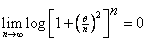,

or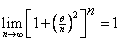,

since the logarithm is continuous.